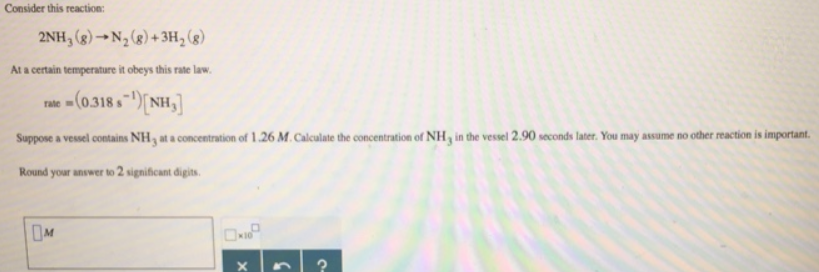# Problem: Consider this reaction:2NH3 (g) → N2 (g) + 3H2 (g) At a certain temperature it obeys this rate rate = (0.318 s-1)[NH3] Suppose a vessel contains NH3 at a concentration of 1.26 M. Calculate the concentration of NH3 in the vessel 2.90 seconds later. You may assume no other reaction is important. Round your answer to 2 significant digits.

###### FREE Expert Solution
80% (80 ratings)###### Problem Details

Consider this reaction:

2NH3 (g) → N2 (g) + 3H(g)

At a certain temperature it obeys this rate rate = (0.318 s-1)[NH3] Suppose a vessel contains NH3 at a concentration of 1.26 M. Calculate the concentration of NH3 in the vessel 2.90 seconds later. You may assume no other reaction is important.